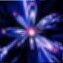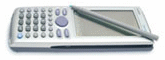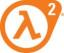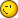# Linear Regresion In Spreadsheed Or Statistics

7 replies to this topic

### #1girdeux

girdeux

•• Members
•• 88 posts
• Location:Spain / Castell?n

• Calculators:
casio fx-115ms;

Posted 15 January 2007 - 07:43 PM

There is any form to do a lineal regresion that pass through origin (point 0,0) in the CP300? With out put many points (0,0).

### #2Lovecasio

Lovecasio

Casio Freak

•• Members
•• 242 posts
• Location:Hochiminh city Vietnam
• Interests:Organic chemistry.<br />Pharmacy

• Calculators:
fx 570 MS, Casio AFX 2.0+, ClassPad 300

Posted 16 January 2007 - 01:31 AM

Sorry, but I don't understand your question. Could you explain more?

### #3Behnoud

Behnoud

Casio Technician

•• Members
•• 338 posts
• Gender:Male
• Location:Iran

• Calculators:
CP 300+ OS2.2/
FX 3600 pv/
FX 115 s/
FX 602P

Posted 16 January 2007 - 05:54 PM

I have this problem as you!
I need Linear reg passing a certain (x,y) not only (0,0).

### #4girdeux

girdeux

•• Members
•• 88 posts
• Location:Spain / Castell?n

• Calculators:
casio fx-115ms;

Posted 16 January 2007 - 07:13 PM

Sorry, but I don't understand your question. Could you explain more?

Ok you imagin that we have some points (x,y) and we want to make a linear regresion with this points, you obtain y = 6,9 x + 9*10^-2 and you know that this function f(x) necessarily for x=0 -> y=0. This function muts pass trought point (0,0).

9*10^-2 it's small value but some times it's better obtain a regresion like: y=a*x; a=cte; with out b (origin of coordinates), I know that it's possible with exel, but with CP?

One solution is add much points (0,0) to the list of regresion, but I look for a more pretty solution.### #5The_AFX_Master

The_AFX_Master

Casio Overlord

•• [Legends]
•• 519 posts
• Gender:Male
• Location:Black Mesa Research Facility (sector C)
• Interests:BASIC +FORTRAN 90+ C++.....and HALF LIFE

• Calculators:
Casio Algebra FX 2.0 Plus, Casio fx 570 ms, Classpad 300, And a crowbar

Posted 16 January 2007 - 10:21 PM

well, that 9e-9 is for sure a floating point small error.. who cares?.. remember that you need N+1 points to perform an n-grade regression.. if you have more points of that N+1, and one point have a small offset, for sure you'll get such small numbers on the B constant. i know that you can enforce a regression to pass over the origin in excel, but afaik.. isn't possible( imho useless because you put errors) on the classpad

Girdeux, you know how least squares method works (minimos cuadrados)?, seems to be you not.... You can read it on a Numerical Methods book (As Chapra-Canale or Burden-Faires). Well, PAP is the pro of the numerical methods here, wait for him### #6PAP

PAP

Casio Overlord

•• Members
•• 681 posts
• Gender:Male
• Location:Somewhere in Europe.
• Interests:Computer Algebra, Numerical Analysis.

• Calculators:
ClassPad 300 (plus an old Casio model, with only a few Kb ram).

Posted 17 January 2007 - 08:04 AM

Well, PAP is the pro of the numerical methods here, wait for himWow, I know something about numerical methods, but I never thought I'm a "pro"....

Ok you imagin that we have some points (x,y) and we want to make a linear regresion with this points, you obtain y = 6,9 x + 9*10^-2 and you know that this function f(x) necessarily for x=0 -> y=0. This function muts pass trought point (0,0).

First of all, I have to say that I don't use built-in linear regression much (in fact, not at all). However, linear regression uses the least squares method in order to obtain a "best" fitting curve f(x)=a*x+b. In other words, you don't have control over the fitting function, so you can't make it pass from 0,0 (b=0). Adding a data point (0,0) will not help in most cases, since the fitting curve don't necessarily pass from a data point.
There is a fast solution, but you need to use CPLua and LNA for this: use my LNA function LMFit, with f(x)=a*x as a model function, instead of f(x)=a*x+b. LMFit will return the correct value for the slope, a, and the fitting curve will pass from 0,0 (not near this point). If using CPLua and LNA is not a problem for you, try it (it's easy, just modify the example program XLMFit). If you have problems, send me your data, and I will post a small CPLua program that uses LNA to do what you want.

### #7The_AFX_Master

The_AFX_Master

Casio Overlord

•• [Legends]
•• 519 posts
• Gender:Male
• Location:Black Mesa Research Facility (sector C)
• Interests:BASIC +FORTRAN 90+ C++.....and HALF LIFE

• Calculators:
Casio Algebra FX 2.0 Plus, Casio fx 570 ms, Classpad 300, And a crowbar

Posted 17 January 2007 - 01:33 PM

@ PAP, at least on the forum, You know more about NM's than us.### #8girdeux

girdeux

•• Members
•• 88 posts
• Location:Spain / Castell?n

• Calculators:
casio fx-115ms;

Posted 22 January 2007 - 08:31 PM

I don't havo much time but I have made a program with CPlua wich calculates the coeficients a,b for y=a+b*x, the R^2 and the coeficient b for y=b*x.

It's all ok, but I get a bad R^2, for lines that cross the origin. I use this ecuations, can someone tell me if they are bad or if I should use other ecuations?

```n = number of points
mx=mean(xi)
my=mean(yi)
mxy=mean(xi*yi)
mx2=mean(xi^2)

sy2=sum( (yi-my)^2 )/n
sxy2=sum( (xi-mx)*(yi-my) )/n
b=mxy/mx2
R^2=b*sxy2/sy2```

#### 0 user(s) are reading this topic

0 members, 0 guests, 0 anonymous users